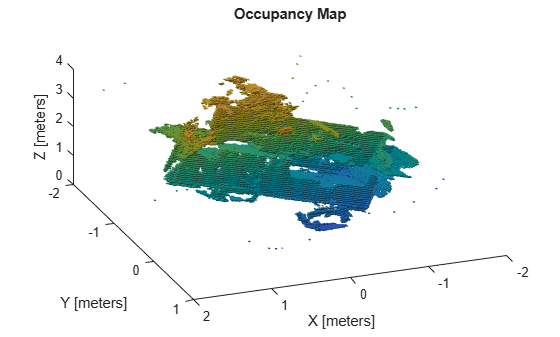Documentation

## Build Occupancy Map from Depth Images Using Visual Odometry and Optimized Pose Graph

This example shows how to reduce the drift in the estimated trajectory (location and orientation) of a monocular camera using 3-D pose graph optimization. In this example, you build an occupancy map from the depth images, which can be used for path planning while navigating in that environment.

### Load Estimated Poses for Pose Graph Optimization

Load the estimated camera poses and loop closure edges. The estimated camera poses were computed using visual odometry. The loop closure edges were computed by finding the previous frame that saw the current scene and estimating the relative pose between the current frame and the loop closure candidate. Camera frames are sampled from a data set that contains depth images, camera poses, and ground truth locations .

```load('estimatedpose.mat'); % Estimated poses load('loopedge.mat'); % Loopclosure edge load('groundtruthlocations.mat'); % Ground truth camera locations ```

### Build 3-D Pose Graph

Create an empty pose graph.

`pg3D = poseGraph3D;`

Add nodes to the pose graph, with edges defining the relative pose and information matrix for the pose graph. Convert the estimated poses, given as transformations, to relative poses as an `[x y theta qw qx qy qz]` vector. An identity matrix is used for the information matrix for each pose.

```len = size(estimatedPose,2); informationmatrix = [1 0 0 0 0 0 1 0 0 0 0 1 0 0 0 1 0 0 1 0 1]; % Insert relative poses between all successive frames for k = 2:len % Relative pose between current and previous frame relativePose = estimatedPose{k-1}/estimatedPose{k}; % Relative orientation represented in quaternions relativeQuat = tform2quat(relativePose); % Relative pose as [x y theta qw qx qy qz] relativePose = [tform2trvec(relativePose),relativeQuat]; % Add pose to pose graph addRelativePose(pg3D,relativePose,informationmatrix); end```

Add a loop closure edge. Add this edge between two existing nodes from the current frame to a previous frame. Optimize the pose graph to adjust nodes based on the edge constraints and this loop closure. Store the optimized poses.

```% Convert pose from transformation to pose vector. relativeQuat = tform2quat(loopedge); relativePose = [tform2trvec(loopedge),relativeQuat]; % Loop candidate loopcandidateframeid = 1; % Current frame currentframeid = 100; addRelativePose(pg3D,relativePose,informationmatrix,... loopcandidateframeid,currentframeid); optimizedPosegraph = optimizePoseGraph(pg3D); optimizedposes = nodes(optimizedPosegraph); figure; show(pg3D);```### Create Occupancy Map from Depth Images and Optimized Poses

Load the depth images and camera parameters from the dataset .

```load('depthimagearray.mat'); % variable depthImages load('freburgK.mat'); % variable K```

Create a 3-D occupancy map with a resolution of 50 cells per meter. Read in the depth images iteratively and convert the points in the depth image using the camera parameters and the optimized poses of the camera. Insert the points as point clouds at the optimized poses to build the map. Show the map after adding all the points. Because there are many depth images, this step can take several minutes. Consider uncommenting the `fprintf` command to print the progress the image processing.

```map3D = occupancyMap3D(50); for k = 1:length(depthImages) points3D = exampleHelperExtract3DPointsFromDepthImage(depthImages{k},K); % fprintf('Processing Image %d\n', k); insertPointCloud(map3D,optimizedposes(k,:),points3D,1.5); end```

Show the map.

```figure; show(map3D); xlim([-2 2]) ylim([-2 1]) zlim([0 4]) view([-201 47])```### References

 Galvez-López, D., and J. D. Tardós. "Bags of Binary Words for Fast Place Recognition in Image Sequences." IEEE Transactions on Robotics. Vol. 28, No. 5, 2012, pp. 1188-1197.